# Where can mirror lines go?

Mirror lines can go anywhere.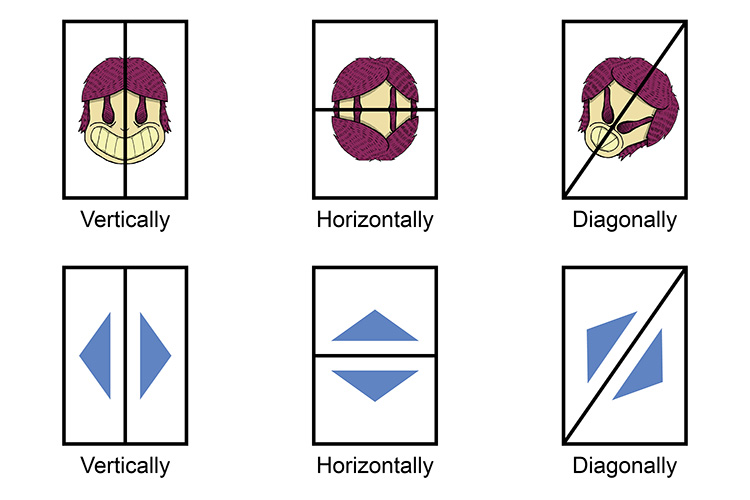Where are the mirror lines?

Remember the formula for a straight line is:

y=mx+c

(you must see and learn the section on straight lines before you proceed.)

## Verticle lines

Example

Draw a reflection of the following image with a mirror line on x=3.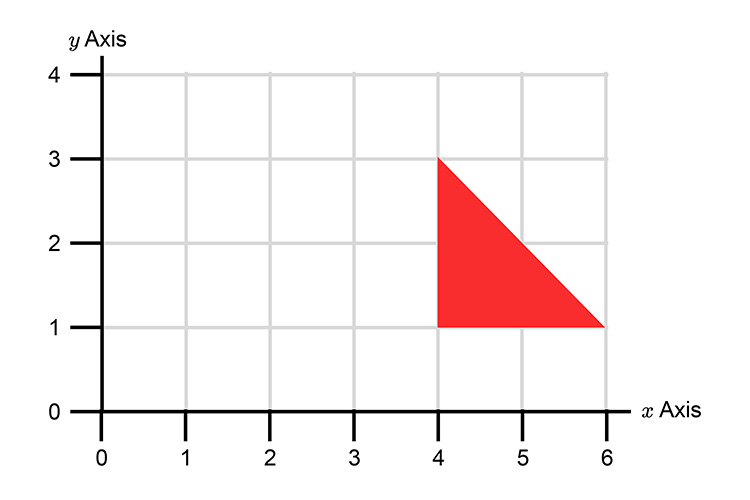NOTE:

For x=3  we can work out that if:

y=1  then x=3

y=2  then x=3

y=3  then x=3  etc

If we plot this on our graph we can find the mirror line.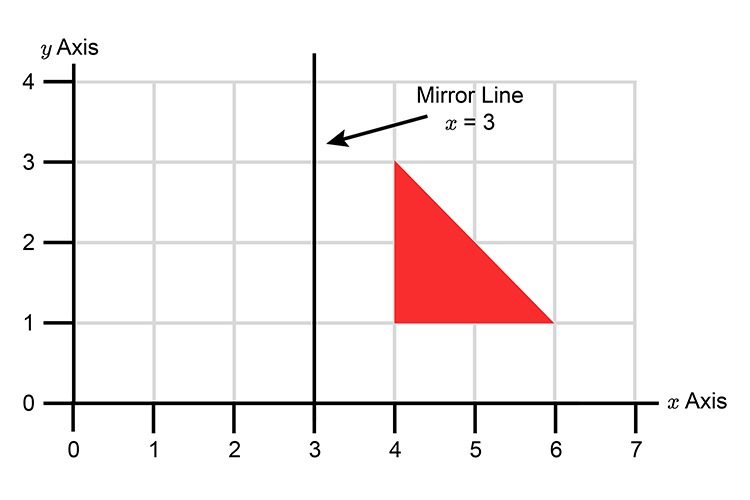We can now complete the reflection.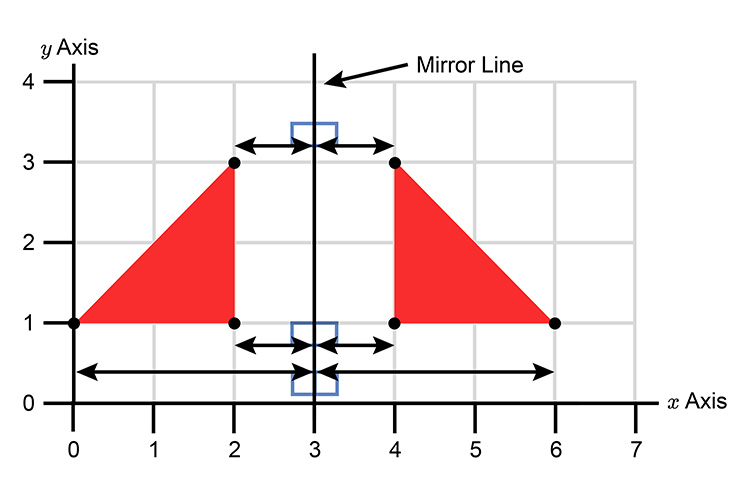## Horizontal lines

Example

Draw a reflection of the following image with a mirror line on y=4.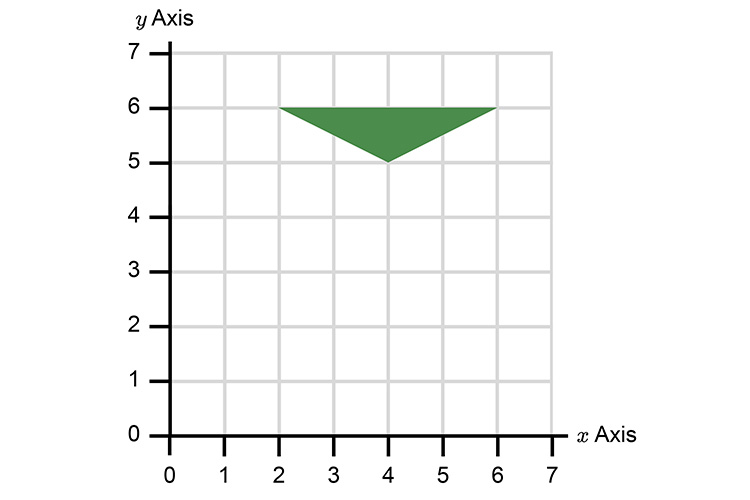NOTE:

For y=4  we can work out that if:

x=5  then y=4

x=4  then y=4

x=3  then y=4  etc

If we plot this on our graph we can find the mirror line.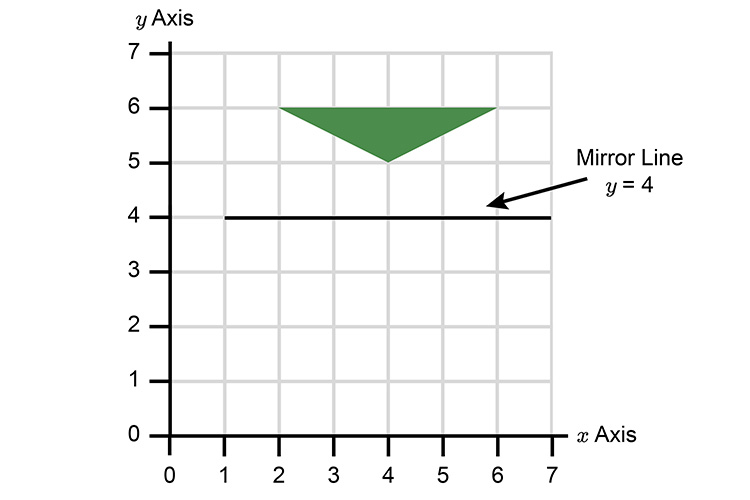We can now complete the reflection.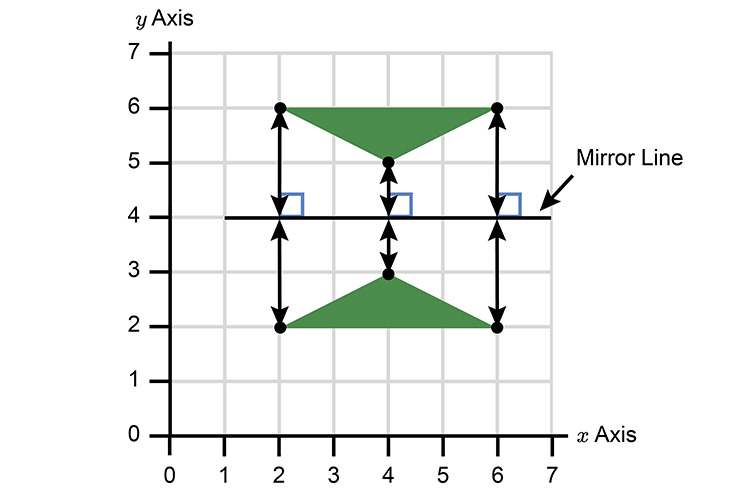## Diagonal lines

Example 1

Draw a reflection of the following image with a mirror line on y=x.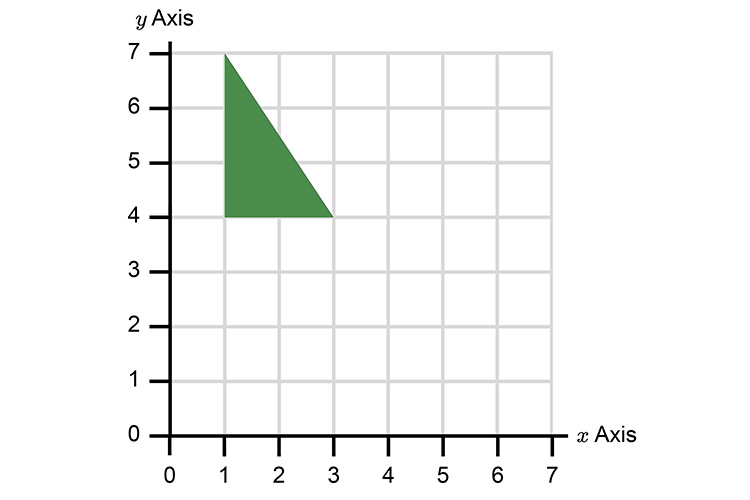NOTE:

For y=x  we can work out that if:

y=1  then x=1

y=2  then x=2

y=3  then x=3  etc

If we plot this on our graph we can find the mirror line.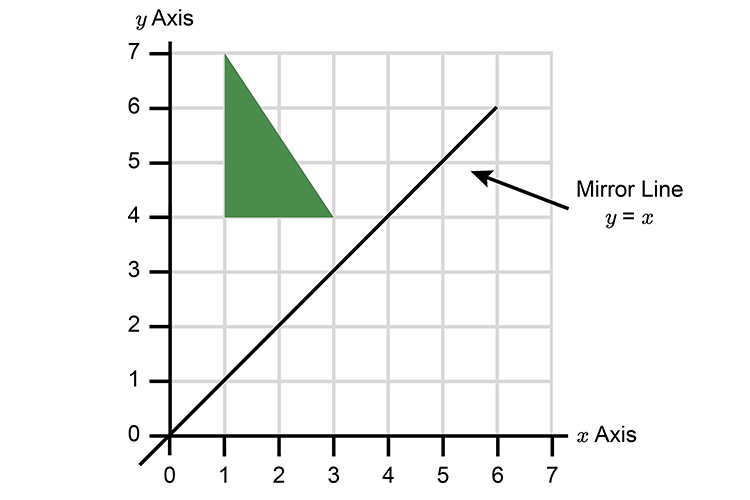We can now complete the reflection.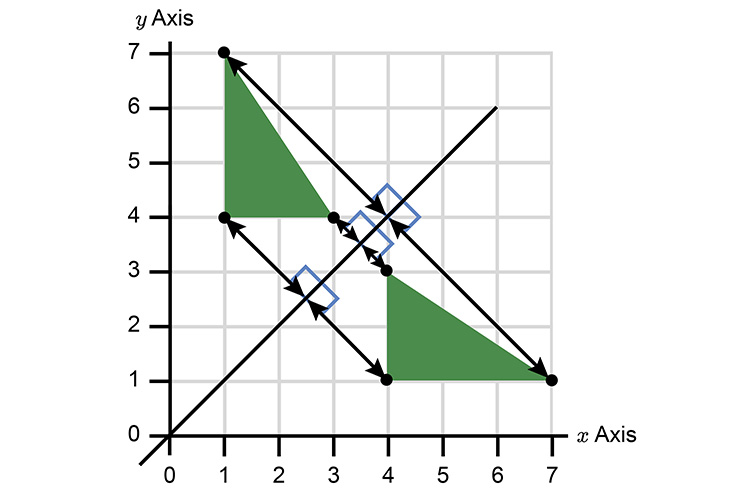Example 2

Draw a reflection of the following image with a mirror line y=-x.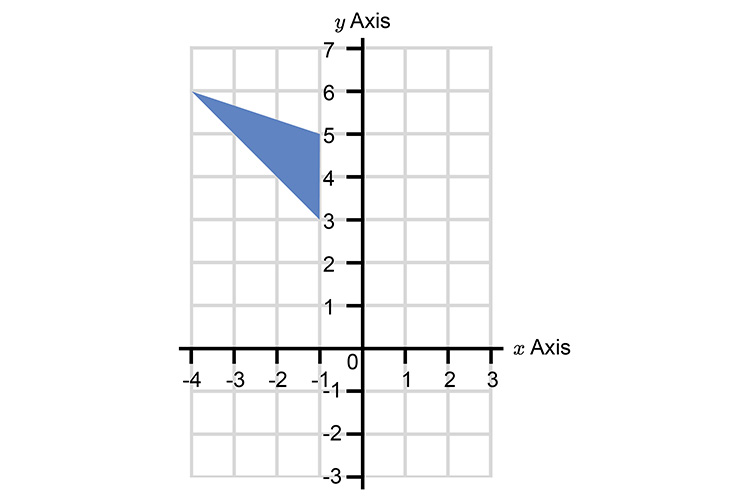NOTE:

For y=-x  we can work out that if:

y=1  then x=-1         (1=-1timesx    therefore   x=-1)

y=2  then x=-2

y=3  then x=-3  etc

If we plot this on our graph we can find the mirror line.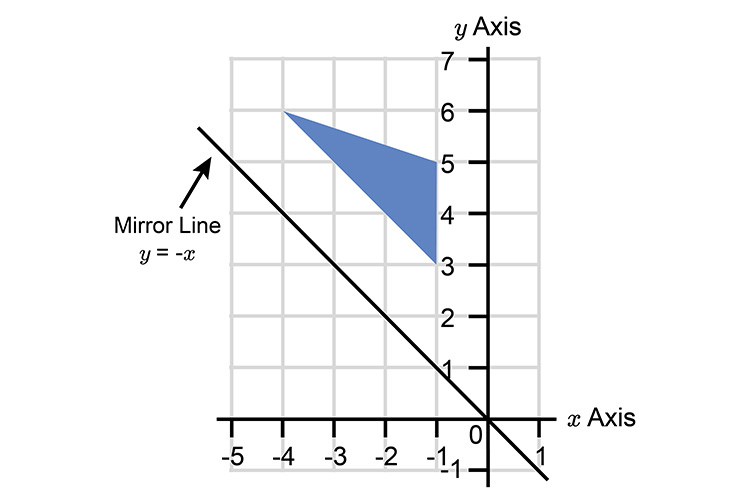We can now complete the reflection.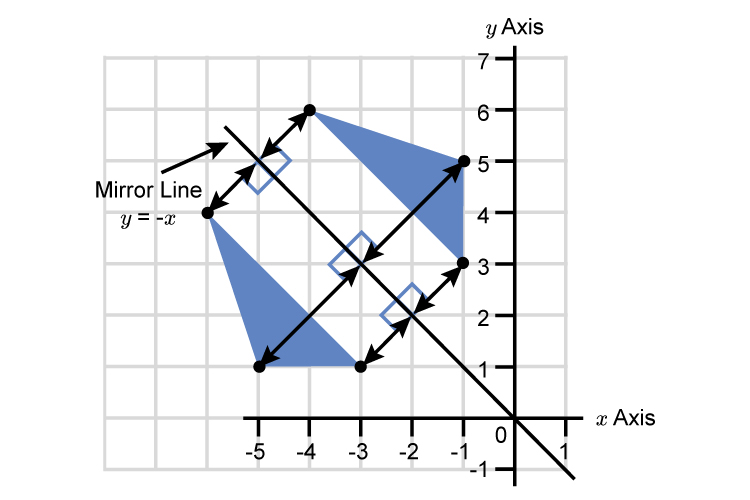## Other examples

Example 1

On the grid below reflect the triangle in the line A, B.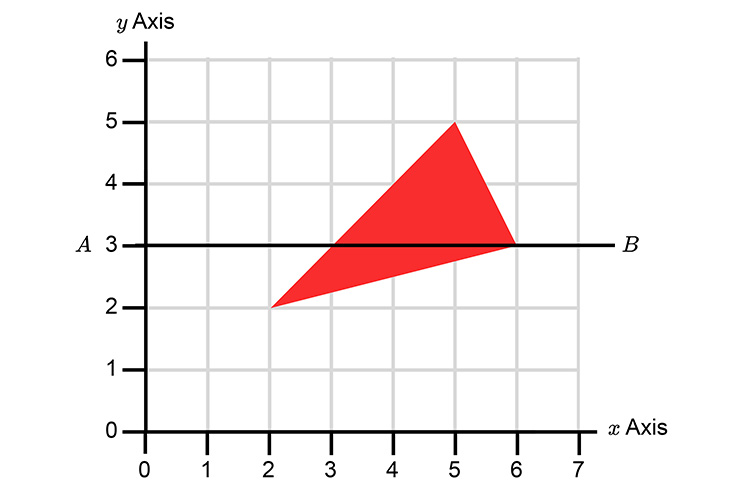Redraw as follows: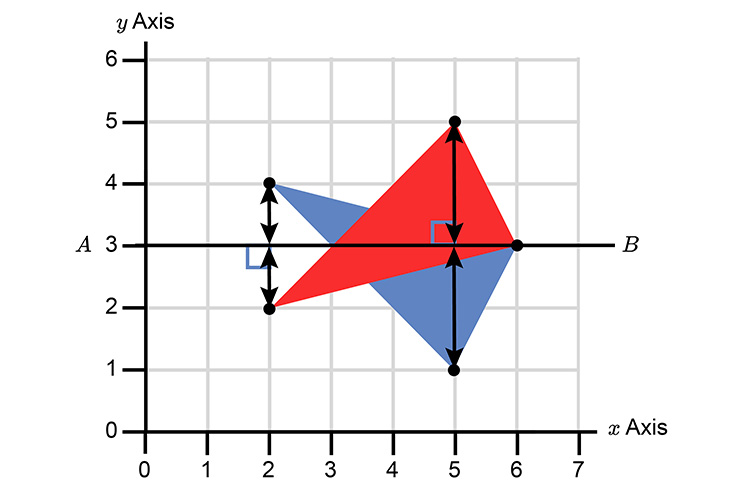Example 2

Describe the single transformation that maps A to B.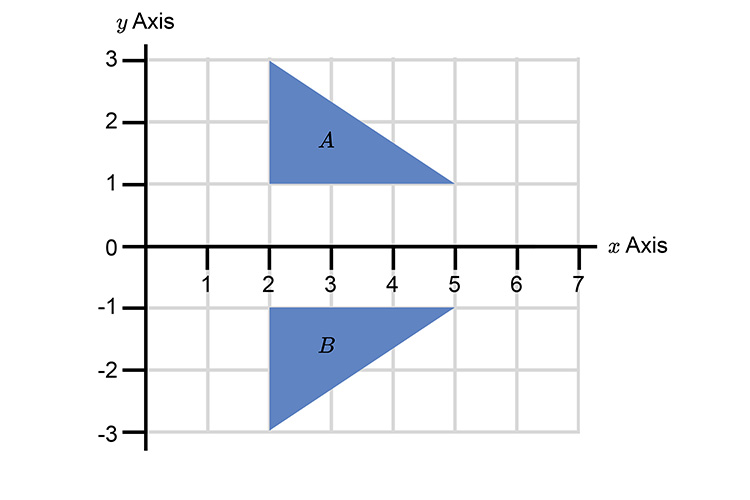You must first describe what this is and it is a REFLECTION.

You must now find the mirror line.

This is easy because we can describe this as a mirror line along the x axis.

Or

If you want to get technical, it is the line y=0.

i.e. x=1  then y=0

x=2  then y=0

x=3  then y=0  etc

This is a reflection where the mirror line is on the x axis.